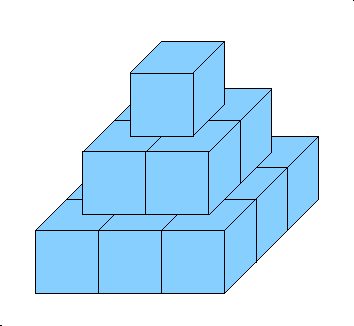### Problem Statement

Consider a N-level pyramid built of unit cubes. An example for N=3 can be seen in the image below.Formally, a pyramid of size N has N levels, where the i-th level (counting from the top) contains an i by i grid of unit cubes.

You have K cubes. First, you select a suitable pyramid size as follows: If K is exactly the number of cubes necessary to build a pyramid of size N for some N, you pick that size. Otherwise, you pick the smallest pyramid size you can not build.

Now you start building the pyramid in a systematic bottom-up way. First you build the complete bottom level, then you build the level above that, etc. When building a level, also proceed in a systematic way, starting the next row only when the previous one is full.

For example, for 21 cubes you should get the following incomplete pyramid:Given an int K specifying the number of cubes you have, return the surface area of the possibly incomplete pyramid you will build according to the instructions above.

### Definition

 Class: PyramidOfCubes Method: surface Parameters: int Returns: double Method signature: double surface(int K) (be sure your method is public)

### Notes

-The returned value must be accurate to within a relative or absolute value of 1E-9.
-The bottom sides of the cubes on the bottommost level are a part of the surface.

### Constraints

-K will be between 1 and 1,000,000,000, inclusive.

### Examples

0)

 `14`
`Returns: 42.0`
 The first example from the problem statement.
1)

 `21`
`Returns: 58.0`
 The second example from the problem statement.
2)

 `1`
`Returns: 6.0`
 A single cube.
3)

 `2`
`Returns: 10.0`
 Two cubes next to each other.
4)

 `451234`
`Returns: 47498.0`
 Quite a lot of cubes.

#### Problem url:

http://www.topcoder.com/stat?c=problem_statement&pm=6577

#### Problem stats url:

http://www.topcoder.com/tc?module=ProblemDetail&rd=9990&pm=6577

misof

#### Testers:

PabloGilberto , brett1479 , Olexiy , marian

#### Problem categories:

Simple Math, Simulation